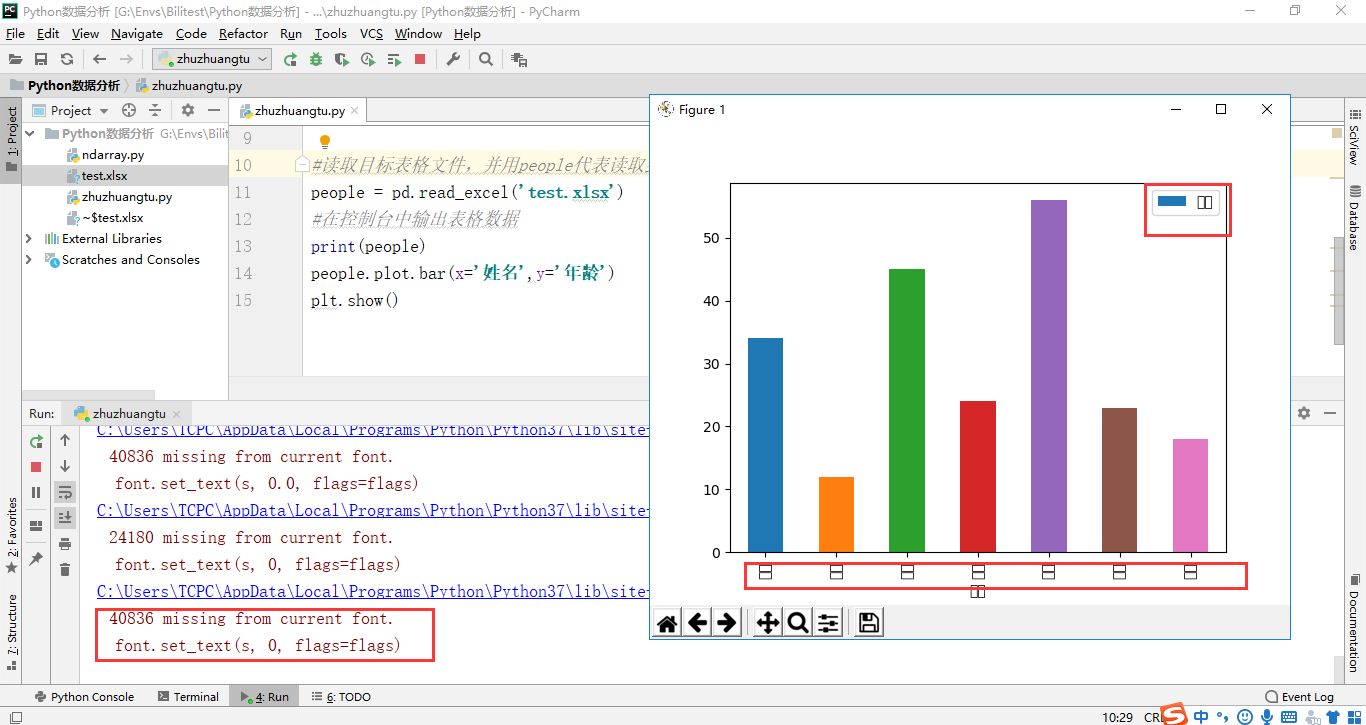• python读取excel制作柱状图和词云图片
千次阅读
2020-11-30 11:50:36

问题描述

需要将excel中的一列内容转换成柱状图和词云，所以用到了matplotlib/xlrd/wordcloud三个库来解决问题

xlrd：读取excel文件

matplotlib：画柱状图

wordcloud：生成词云

具体步骤

xlrd读取文件：

import xlrd

def start_generate():

path = "test.xlsx"

data = xlrd.open_workbook(path)

sheet1 = data.sheet_by_name('Sheet1') # Sheet1 左下角的sheet

list1 = sheet1.col_values(0) # 第一列的数据列表

matplotlib：画柱状图

import matplotlib.pyplot as plt

def draw_from_dict(dicdata, RANGE):

#dicdata：字典的数据。

#RANGE：截取显示的字典的长度。

by_value = sorted(dicdata.items(),key = lambda item:item,reverse=True) # 对字典进行排序

x = []

y = []

for d in by_value:

x.append(d)

y.append(d)

plt.barh(x[0:RANGE], y[0:RANGE]) # 横向柱状图

plt.tight_layout() # 左侧显示不全，使用该函数

plt.savefig('plt.png') # 保存

plt.show()

wordcloud：词云

from wordcloud import WordCloud

def draw_from_list(lis): # 传入的list

text = ' '.join(lis)

wordcloud = WordCloud(background_color='white',

max_words=300,

font_path="Kai.ttf", # 当前目录下中文楷体 结尾有下载

width=1600,

height=1000).generate(text)

wordcloud.to_file("WordCloud.png")

完整代码(完成的是对第一列数据的操作，需要其他列数据自行修改)

import xlrd

import matplotlib.pyplot as plt

from wordcloud import WordCloud

def draw_from_list(lis):

text = ' '.join(lis)

wordcloud = WordCloud(background_color='white',

max_words=300,

font_path="Kai.ttf",

width=1600,

height=1000).generate(text)

wordcloud.to_file("WordCloud.png")

def draw_from_dict(dicdata, RANGE):

#dicdata：字典的数据。

#RANGE：截取显示的字典的长度。

by_value = sorted(dicdata.items(),key = lambda item:item,reverse=True)

x = []

y = []

for d in by_value:

x.append(d)

y.append(d)

plt.barh(x[0:RANGE], y[0:RANGE])

plt.tight_layout()

plt.savefig('test.png')

plt.show()

def start_generate():

path = "test.xlsx"

data = xlrd.open_workbook(path)

sheet1 = data.sheet_by_name('Sheet1')

list1 = sheet1.col_values(0)

dic = dict()

for i in list1:

val = dic.get(i)

if val:

dic[i] = val+1

else:

dic[i] = 1

draw_from_list(list1)

draw_from_dict(dic, len(dic))

if __name__ == '__main__':

start_generate()

遇到的问题

开始生成柱状图的时候，ylable全是乱码，通过这个链接解决了(注意去掉对应配置的'#',最后一步rebuild很重要！)

下载链接

更多相关内容
• 今天小编就为大家分享一篇Python读取Excel表格,并同时折线图和柱状图的方法，具有很好的参考价值，希望对大家有所帮助。一起跟随小编过来看看吧
• Python读取excel文件数据并画柱状图 import xlrd import os import numpy as np import matplotlib.pyplot as plt import math def read_excel(file_path): data = {} workBook = xlrd.open_workbook(file_path...

Python读取excel文件数据并画柱状图

import xlrd
import os
import numpy as np
import matplotlib.pyplot as plt
import math

data = {}

workBook = xlrd.open_workbook(file_path)
allSheetNames = workBook.sheet_names()

for sheet_name in allSheetNames:
temp_data = {}
sheet_content = workBook.sheet_by_name(sheet_name)
rows = sheet_content.nrows
for row in range(rows):
row_data = sheet_content.row_values(row)
if row == 0:
temp_data['type'] = row_data[2:]
else:
temp_data[row_data] = row_data[2:]

data[sheet_name] = temp_data
return data

def show_figure(data, type):

sheet_content = data[type]
num_x = np.arange(len(sheet_content['type']))
fig, ax = plt.subplots()
width = 0.1
num_width = 0
for k in sheet_content:
if k == 'type':
continue
y = sheet_content[k]
for i in range(len(y)):
if y[i] == 'null':
y[i] = 0
y = [i*100 for i in y]
ax.bar(num_x + num_width * width, y, width, alpha=1, label=k)
num_width += 1
ax.set_xticks(num_x + (num_width - 1) * width / 2)
ax.set_xticklabels(sheet_content['type'], fontsize=15)
ax.set_ylabel('MMrTRE [%]', fontsize=15)
#ax.set_title(type, fontsize=25)
ax.legend(fontsize=12, ncol=3)
ax.set_yscale('log')

plt.yticks(fontsize=15)
plt.grid()
plt.show()

if __name__ == '__main__':
repoRoot = os.path.dirname(os.path.realpath(__file__))
file_path = os.path.join(repoRoot, 'ANHIR_metric.xlsx')

# show figure
show_figure(data, 'All')
show_figure(data, 'Evaluation')

展开全文• Python读取Excel表格，同时采用表格中的数值画图柱状图和折线图，这里只需要几行代码便可以实。首先我们需要安装一个Excel操作的库xlrd，这个很简单，在安装Python后直接在DOS命令下输入pip install xlrd,便可以安装...

Python读取Excel表格，同时采用表格中的数值画图柱状图和折线图，这里只需要几行代码便可以实。

首先我们需要安装一个Excel操作的库xlrd，这个很简单，在安装Python后直接在DOS命令下输入pip install xlrd,便可以安装成功，如果还是不行，就输入Python -m pip install xlrd.后面会附上完整的代码和截图：

这行代码就是读取本地Excel文件的：data = xlrd.open_workbook(r'C:\\Users\\ASUS\\Desktop\\txt1\\python3\\yxz2.xlsx')

table = data.sheets()

接下来就是读取列中的数值，也即是列中对象的数据：这个是我举例读取第三列的值table.col_values(2)

我这里采用是pyechats库画图，所以这里还需要安装；pip install pyechats，同时我们需要numpy库中的linspace，等间隔取值，因为Excel中的数据过大是，可能会产生广播尺度超出范围这个尺度。t=np.linspace(1,296,len(y))#等间隔取值

保存到本地HTML格式文件：bar.render(r"C:/Users/ASUS/Desktop/txt1/bokezhexiantu.html")#保存到本地bokezhexiantu.html

以下就是完整代码：# coding:utf-8

#导入读取Excel的库

import xlrd

#导入需要读取Excel表格的路径

data = xlrd.open_workbook(r'C:\\Users\\ASUS\\Desktop\\txt1\\python3\\yxz2.xlsx')

table = data.sheets()

y=''

#将列的值存入字符串

y=table.col_values(2)#读取列的值

#导入pyechats库

from pyecharts import Bar

import numpy as np

t=np.linspace(1,296,len(y))#等间隔取值

bar=Bar("文章阅读量展示","统计如下")#主副标题

bar.show_config()#展示HTML源代码

bar.render(r"C:/Users/ASUS/Desktop/txt1/bokezhexiantu.html")#保存到本地bokezhexiantu.html

结果展示：展开全文• 今天小编就为大家分享一篇Python绘制堆叠柱状图的实例，具有很好的参考价值，希望对大家有所帮助。一起跟随小编过来看看吧
• python 分析表格数据，并绘制成柱状图， 让大家感受一下用python来绘制图表是一种什么感觉。 这是我们今天要分析的表格数据：表格名称为test.xlsx，分为两列，一列是包含姓名信息，一列是包含年龄信息 1、首先...

hello大家好，
我是你们的可爱丸，
我们又见面啦！
python的功能十分强大，
它不仅可以用来做爬虫，
还可以用来做数据分析哦！
那么今天我就带着大家
用python 分析表格数据，并绘制成柱状图，
让大家感受一下用python来绘制图表是一种什么感觉。

这是我们今天要分析的表格数据：表格名称为test.xlsx，分为两列，一列是包含姓名信息，一列是包含年龄信息1、首先我们需要在python中读取表格test.xlsx的数据，并在控制台输出表格数据，检查所选择的表格是否为目标表格

#导入需要用到的模块
import pandas as pd

#读取目标表格文件，并用people代表读取到的表格数据
#在控制台中输出表格数据
print(people)2、将表格数据中的姓名那一列数据设为X轴，年龄那一列数据设为Y轴，并通过plt.show()将柱状图输出

#导入需要用到的模块
import pandas as pd
import matplotlib.pyplot as plt
from matplotlib import rcParams
rcParams['font.family'] = 'simhei'

#读取目标表格文件，并用people代表读取到的表格数据
#在控制台中输出表格数据
print(people)
#x轴是姓名，y轴是年龄
people.plot.bar(x='姓名',y='年龄')
plt.show()3、因为表格数据都为中文，所以在图表中出现了文字乱码的情况，这个时候需要在代码中添加如下中文处理模块，标签文字就会正常显示了。

from matplotlib import rcParams
rcParams['font.family'] = 'simhei'4、虽然标签的乱码已经解决了，但是我们发现：标签方向、数据排列顺序、柱状图颜色都还没有设置好，所以我们可以通过：

• plt.xticks(rotation=360)来调整标签方向
• ascending=False来使数据降序排列，默认为升序排列
• color='blue'将柱状图颜色统一设置为蓝色

注： 对柱状图进行处理后，还需要对inplace参数进行设置，将inplace设置为True才能成功的显示出修改后的表格。

pandas 中 inplace 参数在很多函数中都会有，它的作用是：是否在原对象基础上进行修改
inplace = True：不创建新的对象，直接对原始对象进行修改；
​inplace = False：对数据进行修改，创建并返回新的对象承载其修改结果。

完整代码如下：

# 微信公众号：chimuyhs 【可爱丸学python】 关注并回复：源码  即可获取源代码
# QQ学习交流群：983460742

#导入需要用到的模块
import pandas as pd
import matplotlib.pyplot as plt
from matplotlib import rcParams
rcParams['font.family'] = 'simhei'

#读取目标表格文件，并用people代表读取到的表格数据
#x轴是姓名，y轴是年龄，让直方图排序显示，默认升序
people.sort_values(by='年龄',inplace=True,ascending=False)
#在控制台中输出表格数据
print(people)
#将直方图颜色统一设置为蓝色
people.plot.bar(x='姓名',y='年龄',color='blue')
#旋转X轴标签，让其横向写
plt.xticks(rotation=360)
plt.show()


运行效果如下：好啦，今天的教程就分享到这里啦，如果你觉得有用的话记得点赞、转发支持一下哦~
如果你还想学习更多有关python的学习教程，那就关注我吧，我将定期和大家分享python自学之路上的心得！

展开全文大数据
• 本文主要讲述如何使用Python操作Excel绘制柱形。开发工具，环境PyCharmPython3Office Excel前面我们已经创建好了一张Excel表。现在我们要根据已有的数据，往里面添加柱形。柱形 BarChart参考《Python openpyxl...
• 主要介绍了Python读取Excel数据并生成图表过程解析,文中通过示例代码介绍的非常详细，对大家的学习或者工作具有一定的参考学习价值,需要的朋友可以参考下
• excel import pandas as pd import matplotlib.pyplot as plt ... 柱状图 :param excel_path: :param sheet_name: :return: """ plt.rcParams['font.sans-serif'] = ['SimHei'] # 用来正常显示中文标签
• 实现excel表中柱状图的功能，并按金额排序。引入matplotlib.pyplot模块。下面是用pandas的图，excel中出现中文可能会出现报错。import pandas as pdimport matplotlib.pyplot as pltct_excel=pd.read_excel('F:/...
• python实现Excel可视化柱状图效果图实现代码 学习用python实现对excel的操作，并按照姓名成绩制作一个柱状图 （需要一丢丢的基础） 效果图 案例表格是某学校的成绩 算出成绩总和，提取姓名，成绩。 柱状图效果展示 ...
• 今日给大家分享一个Python读取Excel表格，同时采用表格中的数值画图柱状图和折线图，这里只需要几行代码便可以实。首先我们需要安装一个Excel操作的库xlrd，这个很简单，在安装Python后直接在DOS命令下输入pip ...
• 本篇讲下如何使用纯python代码将excel 中的图表导出为图片。这里需要使用的模块有win32com、pythoncom模块。 网上经查询有人已经写好的模块pyxlchart，具体代码如下： from win32com.client import Dispatch import...
• 今天有朋友叫我同时画柱状图和折线图，我试了一下，发现挺有意思的，所以把我的代码分享出来： 数据读取 import pandas as pd import matplotlib.pylab as plt import seaborn as sns import pylab as mpl %...
• 我在进行Python读取Excel画柱状图和折线图时一直没法解决横轴不能显示表中名字的问题，请大大指导一下！！
• pythonexcel的数据生成柱状图并导出成图片 由于python初学，所以分为两个步骤来进行，1.首先把excel的图表导出成.html文件。2.然后把html文件转换成png文件。其中使用的工具包为pyecharts（绘图），pandas（数据...数据可视化
• python的Pandas模块读取Excel非常方便，可以生成各种图，这不是重点。重点是如何在生成的柱状图上带上值的标识，这个需要费些心思。 这里举一个百分比的例子，比如我有一个表，统计每一年这五个省GDP占全国的比例。 ...数据可视化
• python xlsxwriter模块生成EXCEL柱状图、饼图安装xlsxwriter导入包代码及说明运行结果 安装xlsxwriter pip3 install xlsxwriter 导入包 import xlsxwriter 代码及说明 import xlsxwriter path_Name = 'test.xlsx' #...django 后端
• Excel表操作python操作excel主要用到xlrd和xlwt这两个库，即xlrd是读excel，xlwt是写excel的库。安装xlrdpip install xlrd简单的表格读取import xlrd#读取表格data=xlrd.open_workbook("table.xlsx")#获取表格的...
• 1、实现excel表中柱状图的功能；2、并按照三个月的合计排序。import pandas as pdimport matplotlib.pyplot as pltusers = pd.read_excel('F:/practice/py/practise_09.xlsx')#print(users)users.plot.bar(x='NAME',...
• python提取excel数据形成折线图、柱状图 python提取excel数据形成折线图、柱状图形成折线图形成柱状图 形成折线图 python提取excel数据 下面展示一些 代码块。 1、首先需要import xlrd 2、将数据打开，table = ...matplotlib...